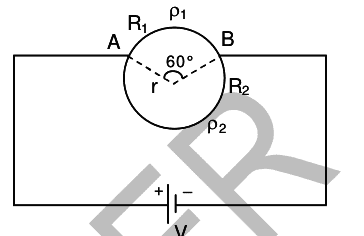# Magnetism w/o calculua

## Homework Statement

In the ATTACHMENT is the given circuit. Which contains two resistance
R1 and R2 in form of circle of radius r = 1 m with a battery
having e.m.f. V = 10∏ volt. Upper resistance is having
resistivity = 4Ω-m & lower resistance having resistivity =
2Ω-m. Angle between two points A and B is 60°. (wires have
same cross section A1 = A2 = 2 cm2)
Find magnitude of magnetic field at centre.

The attempt at a solution
I tried it using the formula B = μiθ/4∏r. BUT THE ANSWER IS NOT COMING CORRECT.